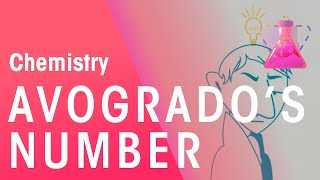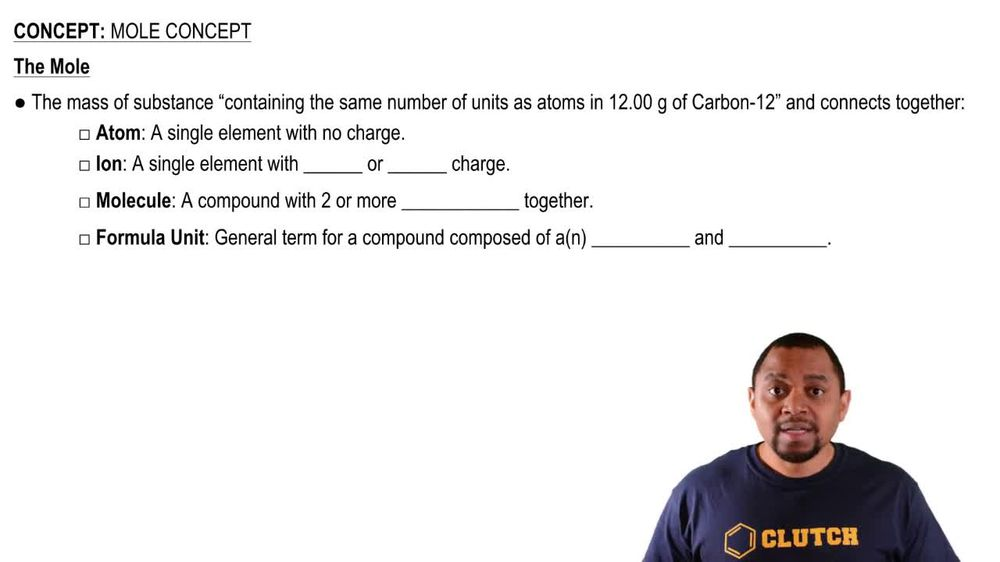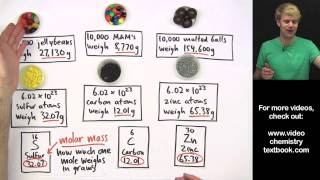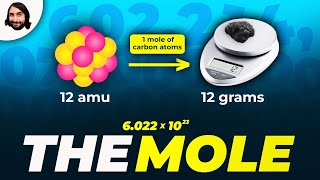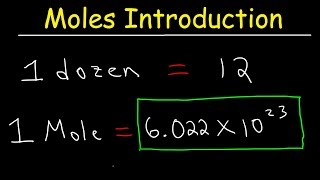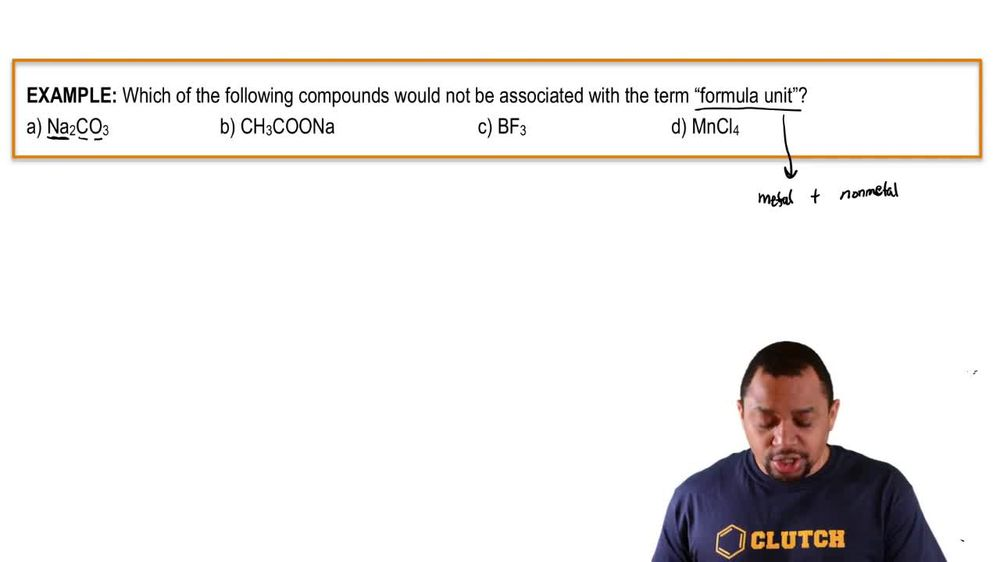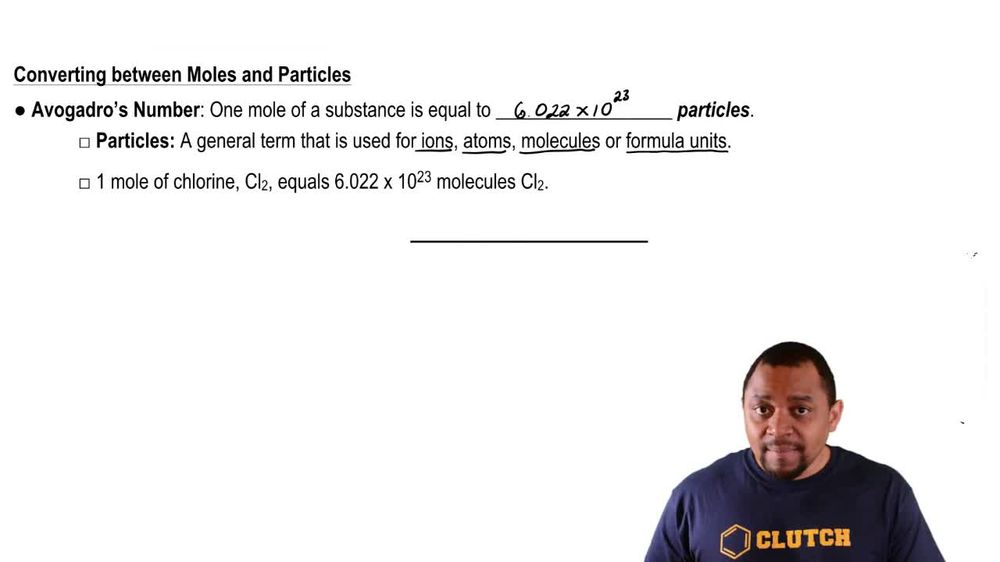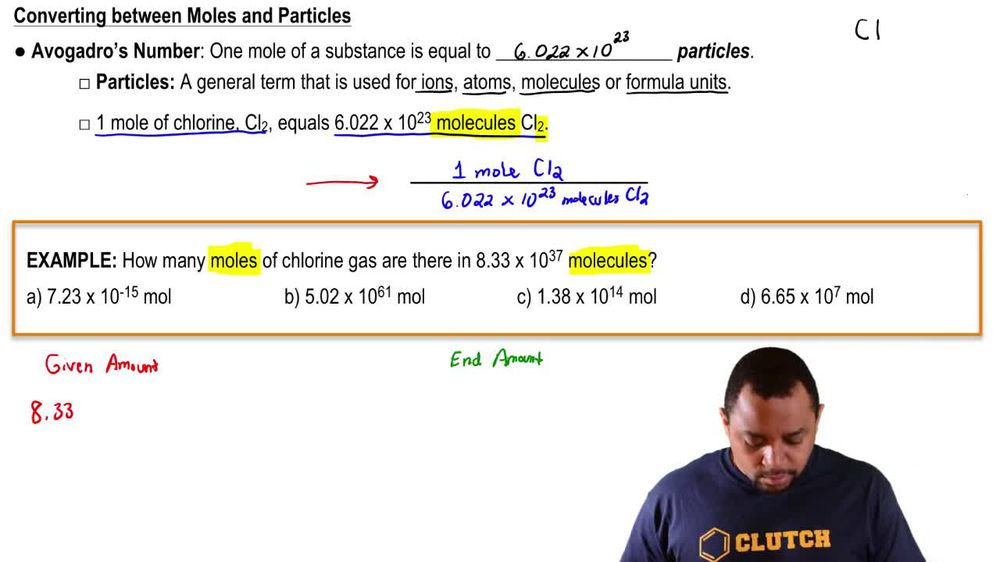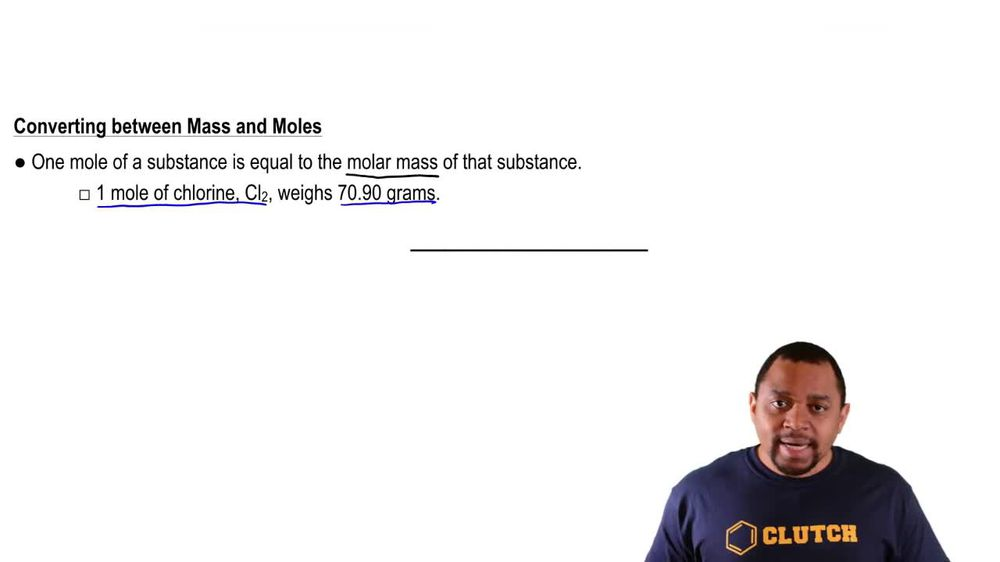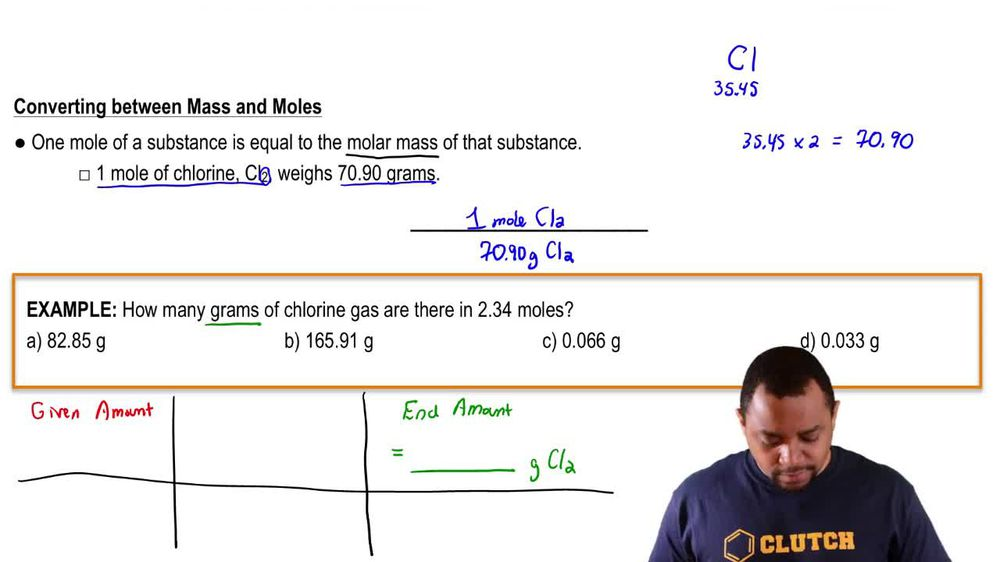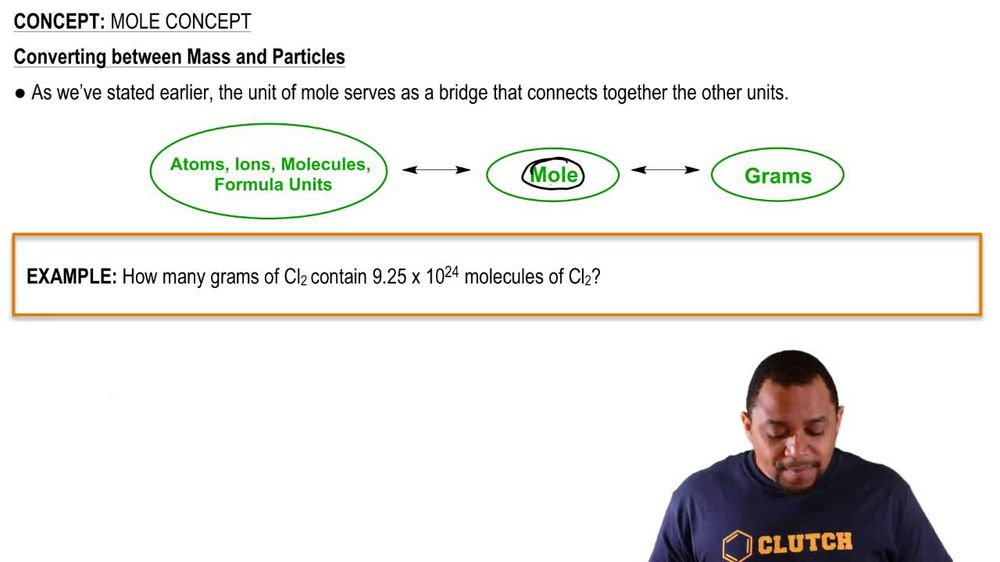Start typing, then use the up and down arrows to select an option from the list.
1. 2. Atoms & Elements2. Mole Concept
Problem

# The “lead” in lead pencils is actually almost pure carbon, and the mass of a period mark made by a lead pencil is about 0.0001 g. How many carbon atoms are in the period?

Relevant Solution1m
Play a video:
Hello everyone today. We have the following question. The mass of a single pencil lead is .041g. If the lead is made entirely out of carbon, calculate the number of carbon atoms in the lead. So first, it's important to note the molar mass or M. M of carbon. And so according to the periodic table, One atom of carbon is going to equal 12.01 g per mole secondly to find the number of carbon atoms. We're going to take the weight that we were given 0. g of carbon. We are first going to multiply by the molar mass, One mole of carbon equaling 12.01 g of carbon are units of g. All canceled out. We will then multiply by the conversion factor that one mole of carbon has 6.02 times 10 to the 23rd Adams Arguments of malls will cancel out and we will just be left with our units of Adams. And so after the calculations, we will be left with 2.1 times 10 to the 21st carbon atoms as our final answer. And with that we have answered the question overall. I hope this helped. And until next time